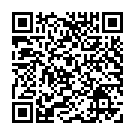## Y3 - Number – Multiplication and Division - Interactive Quiz

### Related Worksheets

An interactive quiz which test your understanding of all the Multiplication and Division objectives in the Year 3 curriculum. Choose one objective or multiple objectives. You can save, or print, your test results as a pdf at the end of the quiz. Ideal for formative or summative assessment.

Alternatively, you can use the Interactive Maths Quiz which includes objectives from all strands of the Year 3 curriculum.

This quiz tests the following objectives:

• recall and use multiplication and division facts for the 3, 4 and 8 multiplication tables
• write and calculate mathematical statements for multiplication and division using the multiplication tables that they know, including for two-digit numbers times one-digit numbers, using mental and progressing to formal written methods
• solve problems, including missing number problems, involving multiplication and division, including positive integer scaling problems and correspondence problems in which n objects are connected to m objectsScan to open this game on a mobile device. Right-click to copy and paste it onto a homework sheet.